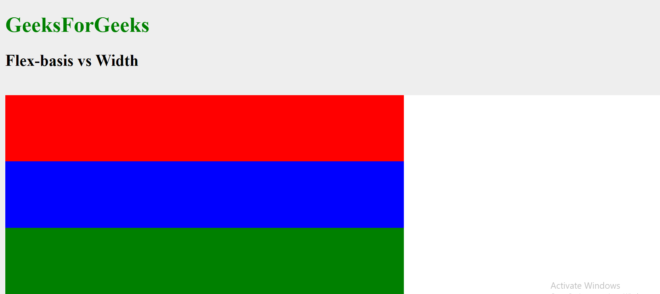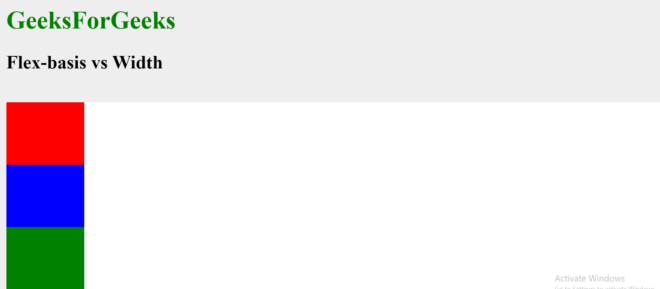# What are the differences between flex-basis and width in Flexbox ?

This article describes the difference between the flex-basis and width property along with examples of usage. The flex-basis property defines the initial size of flex-items depending upon the flex-direction value. It has the following additional properties that can change its behaviour:

• The flex-direction value can be set to row or column. If its value is row, it defines the width and if its value is column, it defines the height.
• The flex-basis property can be applied only to flex-items and width property can be applied to all.
• When using flex property, all the three properties of flex-items i.e, flex-row, flex-shrink and flex-basis can be combined into one declaration, but using width, doing the same thing would require multiple lines of code.
• If flex-direction value is set to be column, then for sizing flex-items horizontally width property is used.

The below examples show the differences between flex-basis and the width and height property:

Example 1: Using flex-basis when flex-direction is set to row.

## HTML

 ` ` `<``html``> ` ` `  `<``head``> ` `    ``<``title``>FlexBox ` `    ``<``style``> ` `        ``body { ` `            ``background: #eee; ` `        ``} ` ` `  `        ``h1 { ` `            ``color: green; ` `        ``} ` ` `  `        ``.wrapper { ` `            ``width: 100%; ` `            ``margin: 0 auto; ` `        ``} ` ` `  `        ``.flex-container { ` `            ``display: flex; ` `            ``background: #fff; ` `            ``flex-wrap: wrap; ` `            ``flex-direction: row; ` `        ``} ` ` `  `        ``.box { ` `            ``/* ` `      ``Since flex-direction is set to ` `      ``row therefore flex-basis defines  ` `      ``the width. Either of the width ` `      ``property or flex-basis property ` `      ``can be used as both perform ` `      ``a similar task in this case. ` `      ``*/ ` ` `  `            ``height: 100px; ` `            ``flex-basis: 600px; ` `        ``} ` ` `  `        ``.one { ` `            ``background: red; ` `        ``} ` ` `  `        ``.two { ` `            ``background: blue; ` `        ``} ` ` `  `        ``.three { ` `            ``background: green; ` `        ``} ` `    `` ` ` ` ` `  `<``body``> ` `    ``<``h1``>GeeksForGeeks ` `    ``<``h2``>Flex-basis vs Width ` `    ``<``br``> ` `    ``<``div` `class``=``"wrapper"``> ` `        ``<``div` `class``=``"flex-container"``> ` `            ``<``div` `class``=``"box one"``> ` `            ``<``div` `class``=``"box two"``> ` `            ``<``div` `class``=``"box three"``> ` `        `` ` `    `` ` ` ` ` `  ` `

Output:Example 2: Using flex-basis when flex-direction is set to column.

## HTML

 ` ` `<``html``> ` ` `  `<``head``> ` `    ``<``title``>FlexBox ` `    ``<``style``> ` `        ``body { ` `            ``background: #eee; ` `        ``} ` ` `  `        ``h1 { ` `            ``color: green; ` `        ``} ` ` `  `        ``.wrapper { ` `            ``width: 100%; ` `            ``margin: 0 auto; ` `        ``} ` ` `  `        ``.flex-container { ` `            ``display: flex; ` `            ``background: #fff; ` `            ``flex-wrap: wrap; ` `            ``flex-direction: column; ` `        ``} ` ` `  `        ``.box { ` `            ``/*  ` `      ``Since flex-direction is set to ` `      ``column therefore flex-basis defines  ` `      ``the height and hence overrides the ` `      ``height property. The height of ` `      ``flex-item will therefore be 80px. ` `      ``The width property has to be separately ` `      ``assigned a horizontal width. ` `      ``*/ ` ` `  `            ``height: 100px; ` `            ``width: 100px; ` `            ``flex-basis: 80px; ` `        ``} ` ` `  `        ``.one { ` `            ``background: red; ` `        ``} ` ` `  `        ``.two { ` `            ``background: blue; ` `        ``} ` ` `  `        ``.three { ` `            ``background: green; ` `        ``} ` `    `` ` ` ` ` `  `<``body``> ` `    ``<``h1``>GeeksForGeeks ` `    ``<``h2``>Flex-basis vs Width ` `    ``<``br``> ` `    ``<``div` `class``=``"wrapper"``> ` `        ``<``div` `class``=``"flex-container"``> ` `            ``<``div` `class``=``"box one"``> ` `            ``<``div` `class``=``"box two"``> ` `            ``<``div` `class``=``"box three"``> ` `        `` ` `    `` ` ` ` ` `  ` `

Output:My Personal Notes arrow_drop_upCheck out this Author's contributed articles.

If you like GeeksforGeeks and would like to contribute, you can also write an article using contribute.geeksforgeeks.org or mail your article to contribute@geeksforgeeks.org. See your article appearing on the GeeksforGeeks main page and help other Geeks.

Please Improve this article if you find anything incorrect by clicking on the "Improve Article" button below.